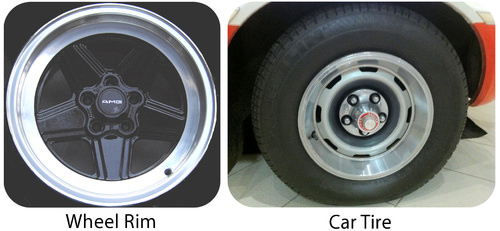# 11.4: Combination Reactions

$$\newcommand{\vecs}{\overset { \rightharpoonup} {\mathbf{#1}} }$$ $$\newcommand{\vecd}{\overset{-\!-\!\rightharpoonup}{\vphantom{a}\smash {#1}}}$$$$\newcommand{\id}{\mathrm{id}}$$ $$\newcommand{\Span}{\mathrm{span}}$$ $$\newcommand{\kernel}{\mathrm{null}\,}$$ $$\newcommand{\range}{\mathrm{range}\,}$$ $$\newcommand{\RealPart}{\mathrm{Re}}$$ $$\newcommand{\ImaginaryPart}{\mathrm{Im}}$$ $$\newcommand{\Argument}{\mathrm{Arg}}$$ $$\newcommand{\norm}{\| #1 \|}$$ $$\newcommand{\inner}{\langle #1, #2 \rangle}$$ $$\newcommand{\Span}{\mathrm{span}}$$ $$\newcommand{\id}{\mathrm{id}}$$ $$\newcommand{\Span}{\mathrm{span}}$$ $$\newcommand{\kernel}{\mathrm{null}\,}$$ $$\newcommand{\range}{\mathrm{range}\,}$$ $$\newcommand{\RealPart}{\mathrm{Re}}$$ $$\newcommand{\ImaginaryPart}{\mathrm{Im}}$$ $$\newcommand{\Argument}{\mathrm{Arg}}$$ $$\newcommand{\norm}{\| #1 \|}$$ $$\newcommand{\inner}{\langle #1, #2 \rangle}$$ $$\newcommand{\Span}{\mathrm{span}}$$$$\newcommand{\AA}{\unicode[.8,0]{x212B}}$$Figure $$\PageIndex{1}$$ (Credit: Rim: User:Relaxatiallc/Wikimedia Commons; Wheel: Christopher Ziemnowicz; Source: Rim: http://commons.wikimedia.org/wiki/File:Ats_amgpenta.jpg(opens in new window); Wheel: http://commons.wikimedia.org/wiki/File:1975_AACA_AMC_Pacer_X_red-white_wheel.jpg(opens in new window); License: Public Domain)

### How useful is a wheel rim?

A wheel rim is not very useful by itself. Driving on the rim can damage it and make for a very rough ride. When the rim is combined with a tire, the product can be put on a car and used for a safe and comfortable ride. The two separate items combine to make something that improves the car ride.

## Combination Reactions

A combination reaction is a reaction in which two or more substances combine to form a single new substance. Combination reactions can also be called synthesis reactions. The general form of a combination reaction is:

$\ce{A} + \ce{B} \rightarrow \ce{AB}\nonumber$

One combination reaction is two elements combining to form a compound. Solid sodium metal reacts with chlorine gas to produce solid sodium chloride.

$2 \ce{Na} \left( s \right) + \ce{Cl_2} \left( g \right) \rightarrow 2 \ce{NaCl} \left( s \right)\nonumber$

In order to write and balance the equation correctly, it is important to remember the seven elements that exist in nature as diatomic molecules: $$\ce{H_2}$$, $$\ce{N_2}$$, $$\ce{O_2}$$, $$\ce{F_2}$$, $$\ce{Cl_2}$$, $$\ce{Br_2}$$, and $$\ce{I_2}$$.

One sort of combination reaction that occurs frequently is the reaction of an element with oxygen to form an oxide. Metals and nonmetals both react readily with oxygen under most conditions. Magnesium reacts rapidly and dramatically when ignited, combining with oxygen from the air to produce a fine powder of magnesium oxide.

$2 \ce{Mg} \left( s \right) + \ce{O_2} \left( g \right) \rightarrow 2 \ce{MgO} \left( s \right)\nonumber$

Sulfur reacts with oxygen to form sulfur dioxide.

$\ce{S} \left( s \right) + \ce{O_2} \left( g \right) \rightarrow \ce{SO_2} \left( g \right)\nonumber$

When nonmetals react with one another, the product is a molecular compound. Often, the nonmetal reactants can combine in different ratios and produce different products. Sulfur can also combine with oxygen to form sulfur trioxide.

$2 \ce{S} \left( s \right) + 3 \ce{O_2} \left( g \right) \rightarrow 2 \ce{SO_3} \left( g \right)\nonumber$

Transition metals are capable of adopting multiple positive charges within their ionic compounds. Therefore, most transition metals are capable of forming different products in a combination reaction. Iron reacts with oxygen to form both iron (II) oxide and iron (III) oxide:

$2 \ce{Fe} \left( s \right) + \ce{O_2} \left( g \right) \rightarrow 2 \ce{FeO} \left( s \right)\nonumber$

$4 \ce{Fe} \left( s \right) + 3 \ce{O_2} \left( g \right) \rightarrow 2 \ce{Fe_2O_3} \left( s \right)\nonumber$

##### Example $$\PageIndex{1}$$: Combination Reactions

Potassium is a very reactive alkali metal that must be stored under oil in order to prevent it from reacting with air. Write the balanced chemical equation for the combination reaction of potassium with oxygen.

###### Step 1: Plan the problem.

Make sure formulas of all reactants and products are correct before balancing the equation. Oxygen gas is a diatomic molecule. Potassium oxide is an ionic compound and so its formula is constructed by the crisscross method. Potassium as an ion becomes $$\ce{K^+}$$, while the oxide ion is $$\ce{O^{2-}}$$.

###### Step 2: Solve.

The skeleton (unbalanced) equation:

$\ce{K} \left( s \right) + \ce{O_2} \left( g \right) \rightarrow \ce{K_2O} \left( s \right) \nonumber\nonumber$

The equation is then easily balanced with coefficients.

$4 \ce{K} \left( s \right) + \ce{O_2} \left( g \right) \rightarrow 2 \ce{K_2O} \left( s \right) \nonumber\nonumber$

Formulas are correct and the resulting combination reaction is balanced.

##### Exercise $$\PageIndex{1}$$

Can you build a balanced, combination reaction for the formation of aluminum oxide (Al2O3)?

4Al + 3O2 → 2Al2O3

Combination reactions can also take place when an element reacts with a compound to form a new compound composed of a larger number of atoms. Carbon monoxide reacts with oxygen to form carbon dioxide, according to the equation:

$2 \ce{CO} \left( g \right) + \ce{O_2} \left( g \right) \rightarrow 2 \ce{CO_2} \left( g \right)\nonumber$

Two compounds may also react to form a more complex compound. A very common example is the reaction of an oxide with water. Calcium oxide reacts readily with water to produce an aqueous solution of calcium hydroxide.

$\ce{CaO} \left( s \right) + \ce{H_2O} \left( l \right) \rightarrow \ce{Ca(OH)_2} \left( aq \right)\nonumber$

Sulfur trioxide gas reacts with water to form sulfuric acid. This is an unfortunately common reaction that occurs in the atmosphere in some places where oxides of sulfur are present as pollutants. The acid formed in the reaction falls to the ground as acid rain.

$\ce{SO_3} \left( g \right) + \ce{H_2O} \left( l \right) \rightarrow \ce{H_2SO_4} \left( aq \right)\nonumber$Figure $$\PageIndex{2}$$: Acid rain has severe consequences on both natural and manmade objects. Acid rain degrades marble statues like the one on the left (A). The trees in the forest on the right (B) have been killed by acid rain. (Credit: (A) Nipik; (B) Nino Barbieri; Source: (A) http://commons.wikimedia.org/wiki/File:Acid_rain_woods1.JPG(opens in new window); (B) http://commons.wikimedia.org/wiki/File:Pollution_-_Damaged_by_acid_rain.jpg(opens in new window); License: (A) Public Domain; (B) CC ​​​​​​​by 2.5(opens in new window))

## Summary

• Combination reactions occur when two or more substances combine to form a new substance.

## Review

1. What are combination reactions?
2. Write the product of the following reaction: Mg+H2O→